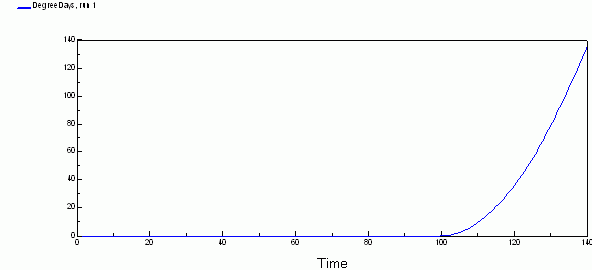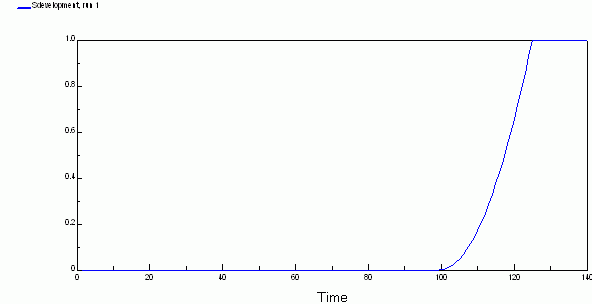# physiological

## Simple degree-days model of temperature influence on development of plants and invertebrates

ModelId:
DegreeDays1
SimileVersion:
4.0

The development rate of plants and invertebrate animals often depends on amount of heat received while the ambient temperature is between minimum and maximum thresholds.

The number of degree-days is a commonly used statistic related to the development of plants and invertebrates. Degree-days, DD, is given by:

DD = ∫ Tt.dt

• T is temperature in degrees Celsius (could be Fahrenheit)
• t is time in days.

degree-days is then oC days or oF days.

Equations:

Compartment DegreeDays : Degree days

Initial value = 0
Rate of change = + dDt on dt
Also called temperature sum or less accurately "heat sum".

Flow dDt on dt : Rate of accumation of degree-days (degC)
dDt on dt = max(T_m-T_thresh,0)
Where:
T_m=../Environment/T_m

Variable Sdevelopment : State of development
Sdevelopment = min(DegreeDays/DDreq,1)
Value = 0 to 1

Equations in Environment

Variable T_m : Mean daily temperature

Results: